# 农业面源污染的环境库兹涅茨拟合曲线验证与分析——以中国西北地区某省为例Verification and Analysis of Environmental Kuznets Fitting Curve of Agricultural Non-Point Source Pollution—A Province in Northwest China Is Taken as an Example

• 全文下载: PDF(1798KB)    PP.362-373   DOI: 10.12677/SD.2019.93044
• 下载量: 205  浏览量: 301

In order to have a more comprehensive understanding of the characteristics of agricultural non-point source pollution in a province in northwest China, the application intensity of fertilizer, the application intensity of pesticide, the density of agricultural film, the total discharge of chemical pollutants in livestock and poultry breeding, and the annual domestic garbage of rural residents were selected as the main evaluation indexes of agricultural non-point source pollution. Combined with the first national census data of pollution sources, five agricultural non-point source pollution indexes were calculated based on the basic data of statistical yearbook. The results show that the overall trend of agricultural non-point source pollution is increasing year by year. From the EKC fitting curve test of agricultural non-point source pollution and per capita agricultural, forestry, animal husbandry and fishery output value, it can be seen that the intensity of fertilizer application, the density of agricultural film use and per capita agricultural, forestry, animal husbandry and fishery output value have a significant inverted u-shaped relationship. There was a positive n-type relationship between the total discharge of chemical pollutants and the per capita output value of agriculture, forestry, animal husbandry and fishery. However, the intensity of pesticide application, the total annual household garbage of rural residents and the per capita output value of agriculture, forestry, animal husbandry and fishery showed a linear increase, which did not conform to the Kuznets curve. Combined with the results of empirical analysis, the paper puts forward some suggestions and measures to improve the situation of non-point source pollution in agriculture, increase agricultural economic growth and promote coordinated green development of agricultural environmental protection.

1. 引言

2. 材料与研究方法

2.1. 研究区概况

2.2. 指标选择与数据来源

2.3. 数据处理方法

E1化肥施用强度(kg/hm2) = 折纯后的化肥施用总量(万t)/耕地总面积(千hm2)；

E2农药施用强度(kg/hm2) = 农药施用总量(万t)/耕地总面积(千hm2)；

E3地膜施用密度(kg/hm2) = 地膜施用量(万t)/地膜覆盖面积(千hm2)；

E5农村居民年生活垃圾量(万t) = 年末乡村人口数(万人) × 0.80 kg/人·d × 365 d。Table 1. Excretion coefficient of all kinds of livestock and poultry feces

Continued

2.4. 模型构建

${E}_{i}={\beta }_{0}+{\beta }_{1}\text{RNGDP}+{\beta }_{2}{\text{RNGDP}}^{2}+{\beta }_{3}{\text{RNGDP}}^{3}+{\mu }_{i}$

${\beta }_{1}$ ≠ 0， ${\beta }_{2}$ = 0时，说明Ei与RNGDP之间呈线性关系；当 ${\beta }_{1}$ > 0， ${\beta }_{2}$ = 0时，说明Ei与RNGDP之间呈线性上升关系；当 ${\beta }_{1}$ < 0， ${\beta }_{2}$ = 0时，说明Ei与RNGDP之间呈线性下降关系；当 ${\beta }_{1}$ < 0， ${\beta }_{2}$ > 0， ${\beta }_{3}$ = 0时，说明Ei与RNGDP之间呈正“U”型；当 ${\beta }_{1}$ > 0， ${\beta }_{2}$ < 0， ${\beta }_{3}$ = 0时，说明Ei与RNGDP之间呈倒“U”型；当 ${\beta }_{1}$ > 0， ${\beta }_{2}$ < 0， ${\beta }_{3}$ > 0时，说明Ei与RNGDP之间呈正“N”型；当 ${\beta }_{1}$ < 0， ${\beta }_{2}$ > 0， ${\beta }_{3}$ < 0时，说明Ei与RNGDP之间呈倒“N”型。

3. 新疆地区农业面源污染与农业经济增长的实证分析

3.1. 新疆农业面源污染的增长趋势分析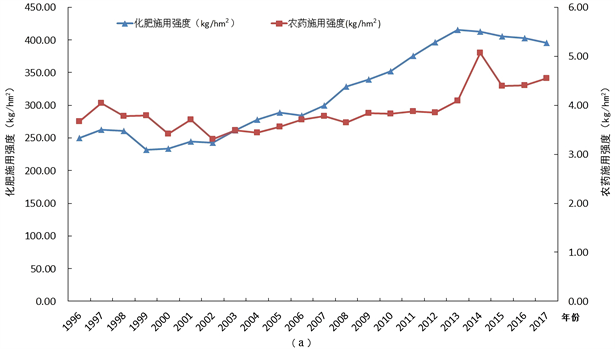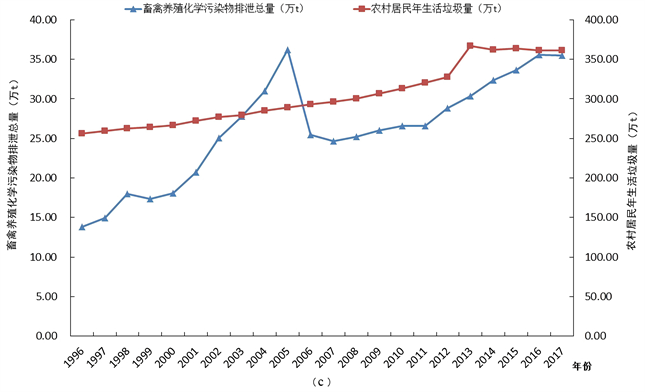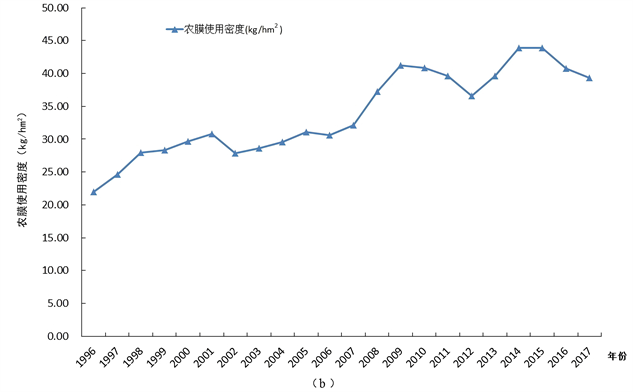Figure 1. Growth trend of indicators of agricultural non-point source pollution in Xinjiang from 1996 to 2017

3.2. 新疆地区农业面源污染物与农业经济增长之间的EKC拟合验证Table 2. OLS regression results of agricultural non-point source pollution indicators and per capita agricultural, forestry, animal husbandry and fishery output value

3.2.1. 化肥施用强度与人均农林牧渔产值EKC拟合分析

${E}_{1}=159.2337-0.0178\text{RNGDP}-3.09\ast {10}^{-7}{\text{RNGDP}}^{2}+{\mu }_{i}$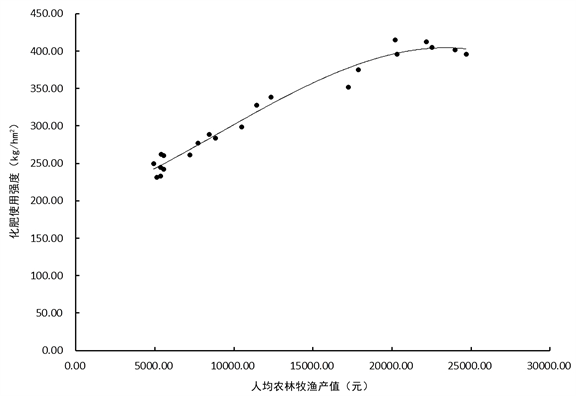Figure 2. Fitting condition of fertilizer application intensity

3.2.2. 农药施用密度与人均农林牧渔产值的EKC拟合分析

${E}_{2}=3.3217+4.46\ast {10}^{-5}\text{RNGDP}+{\mu }_{i}$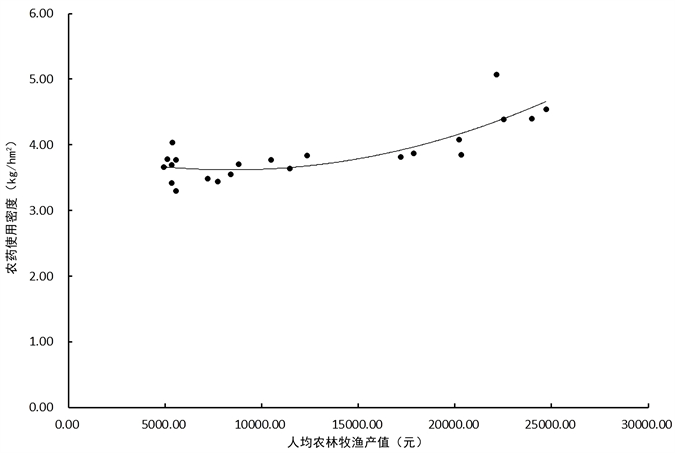Figure 3. Fitting of pesticide application density

3.2.3. 农膜使用密度与人均农林牧渔产值的EKC拟合分析

${E}_{3}=14.5867+0.0026\text{RNGDP}-6.48\ast {10}^{-8}{\text{RNGDP}}^{2}+{\mu }_{i}$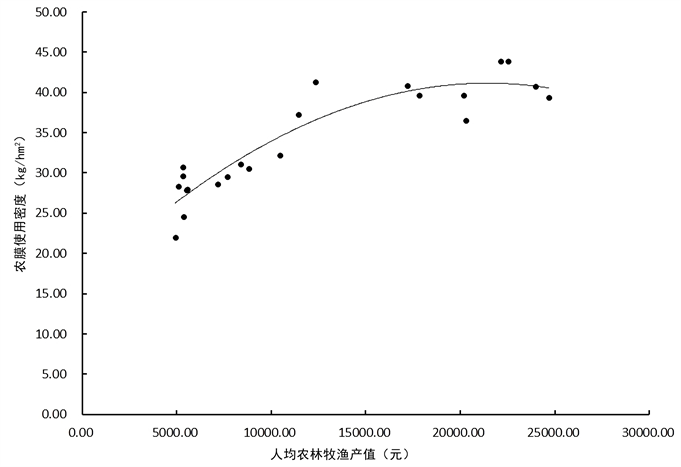Figure 4. Fitting of density of agricultural film use

3.2.4. 畜禽养殖化学污染物排泄总量与人均农林牧渔产值的EKC拟合分析

${E}_{4}=-15.7299+0.0098\text{RNGDP}-6.91\ast {10}^{-7}{\text{RNGDP}}^{2}+1.55\ast {10}^{-11}{\text{RNGDP}}^{3}+{\mu }_{i}$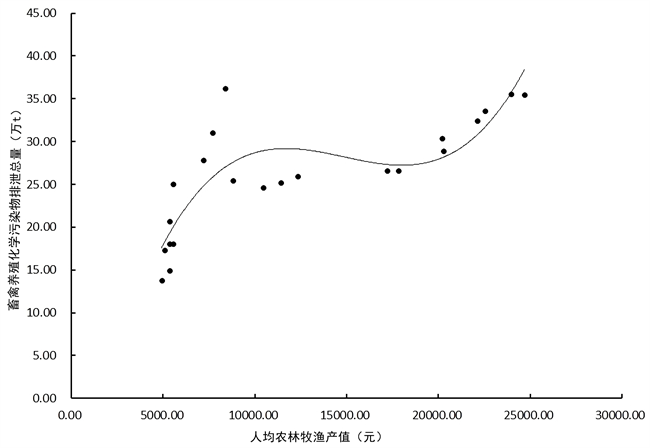Figure 5. Fitting situation of total discharge of chemical pollutants in livestock and poultry breeding

3.2.5. 农村居民生活垃圾总量与人均农林牧渔产值的EKC拟合分析

${E}_{5}=240.4916+0.0051\text{RNGDP}+{\mu }_{i}$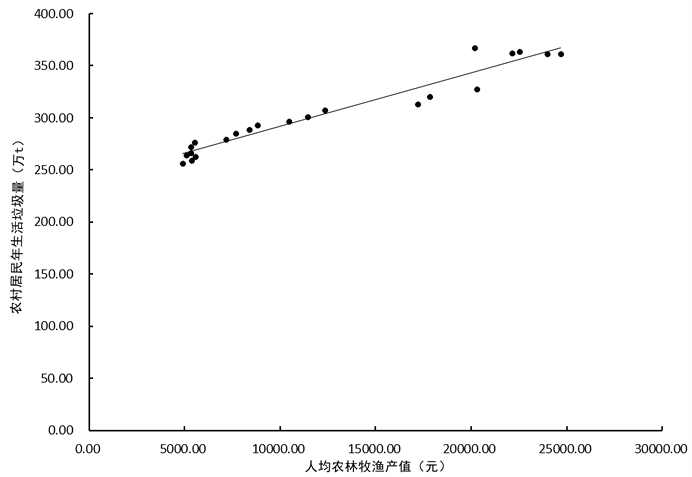Figure 6. Fitting situation of the total amount of household garbage of rural residents

4. 结论及对策

NOTES

*通讯作者。

  习近平. 决胜全面建成小康社会, 夺取新时代中国特色社会主义伟大胜利——在中国共产党第十九次全国代表大会上的报告[J]. 党建, 2017(11): 15-34.  Grossman, G.M. and Krueger, A.B. (1991) Environmental Impacts of a North American Free Trade Agreement. NBER Working Paper Series No. 3914.  吴其勉, 林卿. 农业面源污染与经济增长的动态关系研究——基于1995-2011年福建省数据分析[J]. 江西农业大学学报(社会科学版), 2013, 12(4): 445-452.  史磊, 井晓文. 农业面源污染与农业经济增长关系的实证研究——基于山东省1992-2013年数据的分析[J]. 新疆农垦经济, 2016(1): 8-13.  徐丽萍, 杨其军, 王玲, 等. 新疆地区农业面源污染空间分异研究[J]. 水土保持通报, 2011, 31(4): 150-153+158.  周晓琴, 杨乐, 杨令飞. 新疆农业面源污染物排放量估算及分析[J]. 农业环境科学学报, 2017, 36(7): 1300-1307.  吴义根, 冯开文, 李谷成. 我国农业面源污染的时空分异与动态演进[J]. 中国农业大学学报, 2017, 22(7): 186-199.  谢文宝, 陈彤, 刘国勇. 新疆农业面源污染与农业经济增长的关系——基于脱钩模型和LMDI模型的实证分析[J]. 资源与产业, 2018, 20(1): 68-75.  尚杰, 李新, 邓雁云. 基于EKC的农业经济增长与农业面源污染的关系分析——以黑龙江省为例[J]. 生态经济, 2017, 33(6): 157-160+166.  孟祥海, 周海川, 张俊飚. 中国畜禽污染时空特征分析与环境库兹涅茨曲线验证[J]. 干旱区资源与环境, 2015, 29(11): 104-108.  马国霞, 於方, 曹东, 等. 中国农业面源污染物排放量计算及中长期预测[J]. 环境科学学报, 2012, 32(2): 489-497.